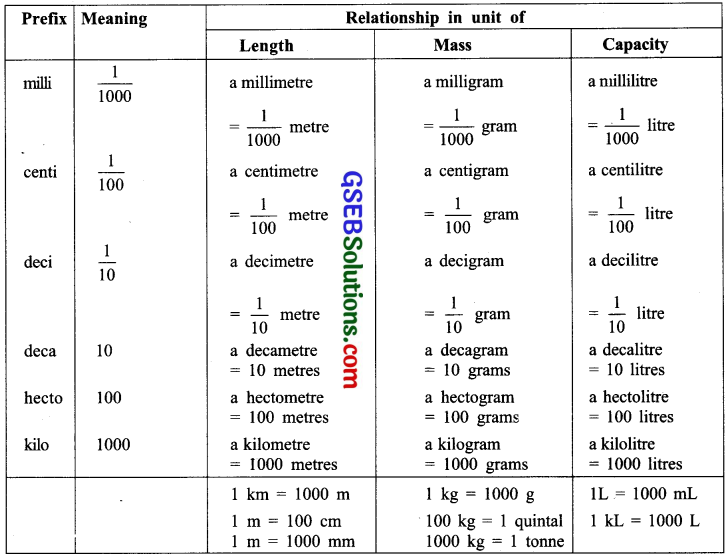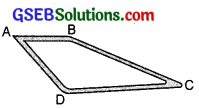# GSEB Solutions Class 7 Maths Chapter 2 Fractions and Decimals Ex 2.5

Gujarat Board GSEB Textbook Solutions Class 7 Maths Chapter 2 Fractions and Decimals Ex 2.5 Textbook Questions and Answers.

## Gujarat Board Textbook Solutions Class 7 Maths Chapter 2 Fractions and Decimals Ex 2.5Question 1.
Which is greater?
(i) 0 0.5 or 0.05
(ii) 0.7 or 0.5
(iii) 1 or 0.7
(iv) 1.37 or 1.49
(v) 2.03 or 2.30
(vi) 0.8 or 0.88
Solution:
(i) Comparing the digits at tenths place, we have 5 > 0
∴ 0.5 > 0.05

(ii) Comparing the digits at tenths place, we have 7 > 5
∴ 0.7 > 0.5

(iii) Comparing the digits at ones place, we have 7 > 0
∴ 7 > 0.7

(iv) Since, the digits at ones place are same.
∴ Comparing the digits at tenths place, we have 3 < 4
∴ 1.37 < 1.49 or 1.49 > 1.37

(v) Since the digits at ones place are same.
∴ Comparing the digits at tenths place, we have 0 < 3
∴ 2.03 < 2.30 or 2.30 > 2.03

(vi) 0.8 can be written as 0.80.
Now, digits at tenths place are same.
∴ Comparing the digits at hundredths place, we have 0 < 8
∴ 0.80 < 0.88 or 0.88 > 0.8Question 2.
Express as rupees using decimals:
(i) 7 paise
(ii) 7 rupees 1 paise
(iii) 77 rupees 77 paise
(iv) 50 paise
(v) 235 paise
Solution:
We know that:
100 paise = ₹ 1 ⇒ 1 paise = ₹ $$\frac { 1 }{ 100 }$$
(i) 7 paise = ₹ 7 x = ₹ $$\frac { 1 }{ 100 }$$ = ₹ 0.07

(ii) 7 rupees 7 paise = ₹ 7 + ₹ 0.07 = ₹ 7.07

(iii) 77 rupees 77 paise= ₹ 77 + 77 paise
= ₹ 77 + ₹ 77 x $$\frac { 1 }{ 100 }$$
= ₹ 77 + ₹ 0.77 = ₹ 77.77

(iv) 50 paise = ₹ 50 x $$\frac { 1 }{ 100 }$$

(v) 235 paise= 200 paise + 35 paise
= ₹ 2 + ₹ 35 x $$\frac { 1 }{ 100 }$$ [∵ 200 paise = ₹ 2]
= ₹ 2 + ₹ 0.35 = ₹ 2.35

Relation between commonly used Measures:Question 3.
(i) Express 5 cm in metre and kilometre
(ii) Express 35 mm in cm, m and km
Solution:
We know that 1 cm = 10 mm, 100 cm = 1 m, 1000 m = 1 km
(i) 5 cm = $$\frac { 5 }{ 100 }$$ m = 0.05 m
5 cm = $$\frac { 5 }{ 100000 }$$ km = 0.00005 km

(ii) 35 mm = $$\frac { 35 }{ 10 }$$ cm = 3.5 cm
35 mm = $$\frac { 35 }{ 1000 }$$m = 0.035 m
35 mm = $$\frac { 35 }{ 100000 }$$ km = 0.000035 km

Question 4.
Express in kg:
(i) 200 g
(ii) 3470 g
(iii) 4 kg 8 g
Solution:
We know that 1000 g = 1 kg
(i) 200 g = $$\frac { 200 }{ 1000 }$$ kg
= $$\frac { 2 }{ 10 }$$ kg
= 0.2 kg

(ii) 3470 g = $$\frac { 3470 }{ 1000 }$$ kg
= 3.470 kg

(iii) 4 kg 8 g = 4 kg + $$\frac { 8 }{ 1000 }$$ kg
= 4 kg + 0.008 kg = 4.008 kgQuestion 5.
Write the following decimal numbers in the expanded form:
(i) 20.03
(ii) 2.03
(iii) 200.03
(iv) 2.034
Solution:
(i) 20.03 = 2 x 10 + 0 x 1 + 0 x $$\frac { 1 }{ 10 }$$ + 3 x $$\frac { 1 }{ 100 }$$
= 2 x 10 + $$\frac { 3 }{ 100 }$$

(ii) 2.03 = 2 x 1 + 0 x $$\frac { 1 }{ 10 }$$ + 3 x $$\frac { 1 }{ 100 }$$
= 2 x 1 + $$\frac { 3 }{ 100 }$$

(iii) 200.03 = 2 x 100 + 0 x 10 + 0 x 1 + 0 x $$\frac { 1 }{ 10 }$$ + 3 x $$\frac { 1 }{ 100 }$$
= 2 x 100 + $$\frac { 3 }{ 100 }$$

(iv) 2.034 = 2 x 1 + 0 x $$\frac { 1 }{ 10 }$$ + 3 x $$\frac { 1 }{ 100 }$$ + 4 x $$\frac { 1 }{ 100 }$$
= 2 x 1 + $$\frac { 3 }{ 100 }$$ + $$\frac { 4 }{ 1000 }$$

Question 6.
Write the place value of 2 in the following decimal numbers:
(i) 2.56
(ii) 21.37
(iii) 10.25
(iv) 9.42
(v) 63.352
Solution:
(i) In 2.56, the digit 2 is at the ones place.
∴ Place value of 2 is 2 x 1, i.e. 2.

(ii) In 21.37, the digit 2 is at the tens place.
∴ Place value of 2 is 2 x 10, i.e. 20.

(iii) In 10.25, the digit 2 is at the tenths place.
∴ Place value of 2 is 2 x $$\frac { 1 }{ 10 }$$, i.e. $$\frac { 2 }{ 10 }$$.

(iv) In 9.42, the digit 2 is at the hundredths place.
∴ The place value of 2 is 2 x $$\frac { 1 }{ 100 }$$, i.e. $$\frac { 2 }{ 100 }$$.

(v) In 63.352, the digit 2 is at the thousandths place.
∴ The place value of 2 is 2 x $$\frac { 1 }{ 100 }$$, i.e. $$\frac { 2 }{ 1000 }$$.Question 7.
Dinesh went A from place A to place B and from there to place C. A is 7.5 km from B and B is 12.7 km from C. Ayub went from place A to place D and from there to place C. D is 9.3 km from A and C is 11.8 km from D. Who travelled more and by how much?Solution:
∵ Distance from A to B = 7.5 km
Distance from B to C = 12.7 km
∴ Distance from A to C through B
= (7.5 + 12.7) km
= 20.2 km
∴ Distance travelled by Dinesh = 20.2 km
Again, Distance from A to D = 9.3 km
Distance from D to C = 11.8 km
∴ Distance from A to C through D
= (9.3 + 11.8) km = 21.1 km
∴ Distance travelled by Ayub = 21.1 km
Since (21.1 – 20.2) km = 0.9 km or 900 m
or 900 m
∴ Ayub travelled more distance by 900 m.

Question 8.
Shyama bought 5 kg 300 g apples and 3 kg 250 g mangoes. Sarala bought 4 kg 800 g oranges and 4 kg 150 g bananas. Who bought more fruits?
Solution:
Since, Shyama bought 5 kg 300 g apples and 3 kg 250 g mangoes,
Total fruits bought by Shyama
= 5 kg 300 g + 3 kg 250 g
= 8 kg 550 g
Since, Sarala bought 4 kg 800 g oranges and 4 kg 150 g bananas,
Total fruits bought by Sarala
= 4 kg 800 g + 4 kg 150 g = 8 kg 950 g
Since, 8 kg 950 g – 8 kg 550 g = 0 kg 400 g
or $$\frac { 400 }{ 1000 }$$ kg = 0.4 kg.
Thus, Sarala bought more fruits by 0.4 kg.Question 9.
How much less is 28 km than 42.6 km?
Solution:
Since 42.6 km – 28 km = 14.6 km
∴ 28 km is less than 42.6 km by 14.6 km.